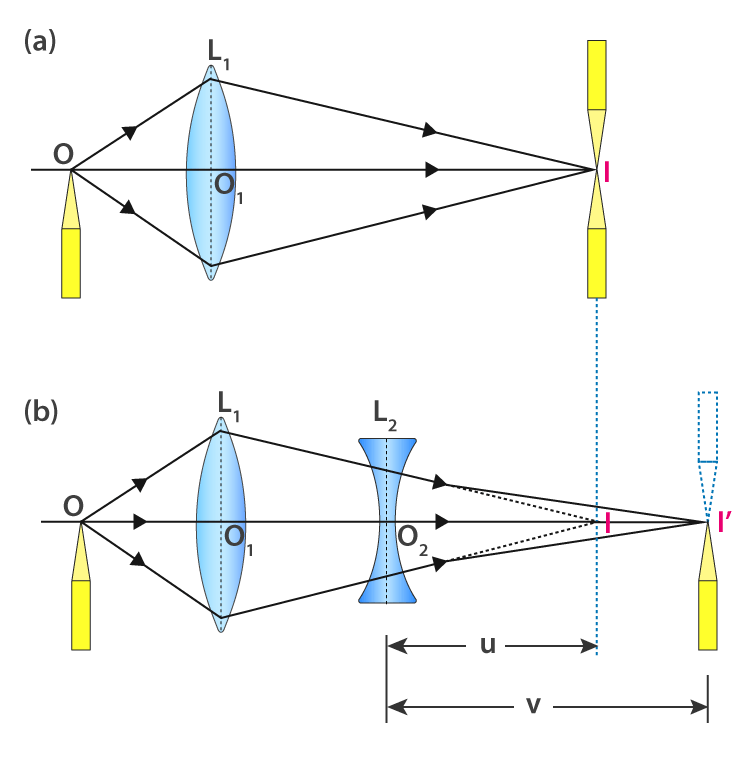# To find the focal length of a concave lens, using a convex lens

## Aim

To find the focal length of a concave lens using a convex lens.

## Materials Required

1. An optical bench with four upright
2. A convex lens with a lens focal length
3. A concave lens with a more focal length
4. Two lens holders
5. One thick and one thin optical needle
6. A knitting needle
7. A half-metre scale

## Theory

We use the lens formula in this experiment to calculate the focal length of the concave lens:

$f=\frac{uv}{u-v}$

Where,

• f is the focal length of the concave lens L1
• u is the distance of I from the optical centre of the lens L2
• v is the distance of I’ from the optical centre of the lens L2.

From sign convention, the f obtained from the above formula will be negative as v > u and u – v is negative.

## Ray Diagram## Procedure

To determine the rough focal length of the convex lens

1. Place the convex lens on the lens holder.
2. Now face the lens towards a distant tree or building.
3. Obtain the image either on the white wall or on a screen and keep moving the lens either forward or backwards till a sharp image is formed.
4. To determine the rough focal length of the lens, measure the distance between the lens and the screen.

To set the convex lens

1. Place the lens on the holder with fixed upright such that the upright is kept at 50 cm mark.
2. The lens should be placed in such a way that its surface is vertical and perpendicular to the length of the optical bench.
3. The upright should be kept in this position throughout.

To set the object needle

1. Place the thin optical needle which is the object needle O near-zero end of the upright which is moveable.
2. Place the object needle upright at a distance nearly 1.5 times the focal length of the lens.
3. The tip of the needle should be horizontal to the optical centre of the lens.
4. Note the position of the index mark below the object needle upright.
5. To see an inverted and enlarged image of the object needle which is in the middle of the lens, close the left eye and see with a right eye open.
6. On the other end of the optical bench, place the image needle on the fourth upright.
7. The tip of the image needle should be in line with the image that is seen with the right eye.
8. To see the parallax, move the eye towards the right. The image needle and object needle are no longer in line.
9. Remove the parallax tip to tip.
10. Note the position of the index mark at the base of the image needle upright.
11. Record the position of the index marks.
12. Now place the concave lens holder on the I side of the convex lens.
13. The upright and convex lens should be placed at a distance from each other.
14. The concave lens should be placed such that it coincides with the principal axes.

To set the image needle at I’

1. Repeat steps 4 and 5.

To get more observations

1. Repeat the experiment by moving the object needle towards the lens by 2cm.
2. Repeat the experiment by moving the object needle away from the lens by 2cm.
3. Record all the observations.

## Observations

The rough focal length of a convex lens = ……….

The actual length of the knitting needle, x = ………

Observed distance between the concave lens and image = ……..

Needle when knitting needle is placed between them, y = ……..

Index correction for u as well as v, x – y = ……..

Table for u, v and f

 Sl. no Position of Observed Corrected $f=\frac{uv}{u-v}$ in cm O in cm L1 at O1 in cm I in cm L2 at O2 in cm I’ in cm u = IO2 in cm v = I’O2 in cm u in cm v in cm 1. f1= 2. f2= 3. f3=

## Calculations

1. To find observed u by finding the difference of position of L2 and I.
2. To find observed v by finding the difference of position of L2 and I’.
3. Corrected values of u and v are obtained by applying index correction.
4. Calculate $f=\frac{uv}{u-v}$.
5. Finding the mean of f
$Mean\,f=\frac{f_{1}+f_{2}+f_{3}}{3}$

## Result

The focal length of the given concave lens = …….. cm

## Precautions

1. The lens must be clean.
2. The focal length of the convex lens should be lesser than the concave lens.
3. For u and v index correction should be applied.
4. To obtain a real and inverted image, the needle should be kept at a certain distance.
5. To avoid parallax, a distance of at least 30 cm should be maintained between the tip of the needle and eye.

## Sources Of Error

1. Vertical uprights might not be used.
2. The removal of parallax might not be perfect.

## Viva Questions

Q1. What is spherical lens?

Ans: Spherical lens is defined as a lens that is part of a sphere and has a surface that is spherical.

Q2. What type of lens is present in human eye?

Ans: Convex lens is present in human eye.

Q3. What are the factors affecting the power of lens?

Ans: Following are the factors affecting the power of lens:

1. Refractive index of the material used in the lens.
2. The change in medium.